# Using model post-processor within scikit-learn pipelines

## On the lack of post-processors¶

Pipelines are a very handy and central feature of the scikit-learn library that enable to chain sequences, or even, in a limited way, DAGs, of data transformers and one machine learning model. They greatly facilitate the application of cross-validation scoring or hyper-parameter search on the resulting pipeline as a whole. They also ease the productization of a machine learning exercise by providing one single encapsulated trained model that can be applied as one instance on a test set or integrated into a production product.

For a very clear introduction, see also the excellent Using scikit-learn Pipelines and FeatureUnions blog post by Zac Stewart.

The two aspects of the Pipeline architecture I want to focus on are:

• each element of the pipeline, except maybe the last one, must be transformers, i.e. provide a transform(X) method
• the last element of a pipeline can either be a transformer, in which case the result behaves like a grand-unified transformer, or a predictor, i.e. a element providing a predict() or predict_proba() (this is a simplification, see the actual API for details). In the later case, the resulting pipeline behaves like a predictor, can be used for scoring and can be used in a cross-validation pipeline.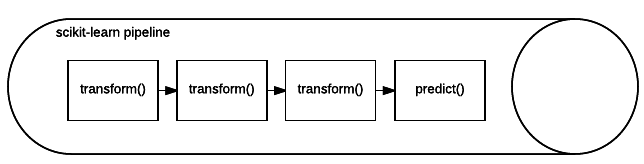I am not knowledgeable about nor experienced with the scikit-learn architecure to understand the rationale of this choice. My naive reaction is that the distinction between the three functions (transform(), predict()and predict_proba() is of importance for other parts of the architecture, or present for historical reasons. My first apporach to this is that maybe just a single transform() function would have been enough and allows more composition flexibility.

A restriction inherent to this design is the difficulty to place transformer after the model. I can think however of several cases where that would come in handy:

• To force a prediction to be within a known validity domain. For example, in this Kaggle tutorial, the output of the model is a count of bike usage events, so it must be integer and non-negative. If I use a model that does not guarantee that (say, a simple linear model), I'd like to be able to pipe the result into a transformer before scoring the result.

• Another (rather theoretical) example is simple composed models, like logistic regression, which stricto-senso is a learning model piped into a sigmoid transformer.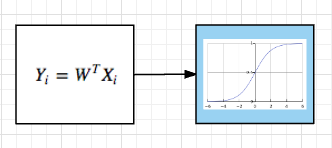• Finall, model calibration techniques, like adjusting predicted probability to actually observed proportion within a validation set, could be implemented as post-processors.

## Existing workarounds¶

Before introducing the simple wrappers I coded below, let's clarify that those "limitation" are not a showstopper.

In the Kaggle example I gave above, an easy workaround consists in writting a custom scoring method that post-processes the model output before actually scoring it. This enables to have a correct score in each fold of the cross-validation loop: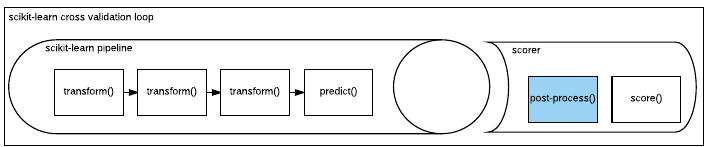In the case of logistic regression, Scikit-learn is of course providing an implementation that includes the sigmoid as art of the model itself, this is working perfectly fine and poses no issues. My only point here is that if one was to re-implement a logistic regression algorithm today, this person would not be able to break it down as a several steps of a scikit-learn pipeline.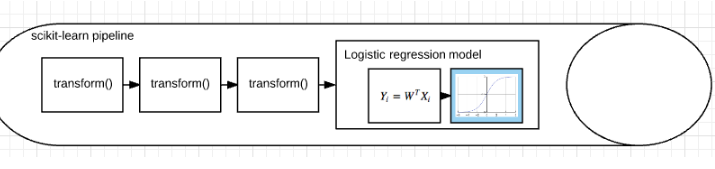In both cases, in term of software design we can see that the concept of transformation leaks from the transformer itself to the nearest adjacent elements in the pipeline, either the scoring method or the model itself.

## Allowing models anywhere in the pipeline¶

A more general solution is to wrap the model such that it behaves like a tranformer. I'm not the author of this idea, some places where it has been hinted before are here and here, among others.

The class below achieves that very simply, by re-routing calls to transform to the underlying pipeline predict():

In [ ]:
from sklearn.pipeline import Pipeline

class TransformingPredictorPipeline(Pipeline):
"""
This allows to convert a predicting pipeline into a transforming pipeline.

This seemed to work when I tested it on scikit-learn 0.18, but you'd rather not believe me.
"""

def __init__(self, steps):
Pipeline.__init__(self, steps)

def transform(self, X):
return self.predict(X)

def fit_transform(self, X, y=None, **fit_params):
return self.fit(X, y, **fit_params).predict(X)


The converse transformation is also required: once we have transformed our prediction model into a transformer and appended some post-processor, we end up with a transformer pipeline. What we want though is a predicting pipeline, with a predict() method, so that we can use it with a scoring method within a cross-validation loop.

In [ ]:
from sklearn.pipeline import Pipeline

class PredictingTransformerPipeline(Pipeline):
"""
This is the reverse conversion of the above: we transform a transforming pipeline
into a predicting pipeline.

This seemed to work when I tested it on scikit-learn 0.18, but you'd rather not believe me.
"""

def __init__(self, steps):
Pipeline.__init__(self, steps)

def predict(self, X):
return self.transform(X)

def fit_predict(self, X, y=None, **fit_params):
return self.fit(X, y, **fit_params).transform(X)


I realize those are quite invaluable snippets of code (ahem...), so not only am I blogging about them but also pushing them to my data toolkit on github.

# Example¶

Using the Kaggle tutorial example mentioned above, let's use a simple linear model to predict the event counts. An obvious issue is that the raw model outputs will be real values with no guarantee of being positive.

A set of simple transformers to fix that are quickly written:

In :
import sys
import numpy as np
from sklearn.base import TransformerMixin, BaseEstimator

class NonNegativeTransformer(TransformerMixin, BaseEstimator):

def transform(self, X, y=None):
"""
Replaces any negative value with its closest known valid value: 0
"""

return np.clip(X, a_min=0, a_max=sys.maxint)

def fit(self, X, y=None):
return self

class AsIntegerTransformer(TransformerMixin, BaseEstimator):

def transform(self, X, y=None):
"""
Replaces any non integer value with its closest known valid value
"""

return np.round(X).astype(int)

def fit(self, X, y=None):
return self



This allows write a pipeline as follows (I like to tell myself that this is very readable):

In [ ]:
from sklearn.preprocessing import StandardScaler
from sklearn.pipeline import Pipeline, FeatureUnion
from sklearn.decomposition import PCA
from sklearn.linear_model import ElasticNet

# name of one-hot encoded nominal features
nominal_enc_features = [
"season_fall", "season_spring", "season_summer", "season_winter",
"weather_clear", "weather_cloudy", "weather_misty"]

# name of numerical features
num_features = ["temp", "humidity", "windspeed", "hourly_dist"]

the_pipe = PredictingTransformerPipeline([
("main_learning_pp", TransformingPredictorPipeline([
("1d-pre-process", FeatureUnion([
("nominal_feat_passthrough", Columns_Selector(nominal_enc_features)),
("numerical_feat_encoding", Pipeline([
("num_cols", Columns_Selector(num_features)),
("scaler", StandardScaler())])
)])
),
("pca", PCA()),
("lr", ElasticNet())])
),
("to_integer", AsIntegerTransformer()),
("to_non_negative", NonNegativeTransformer()),
])


main_learning_pp is really just a very basic pipeline, with one 1d-pre-proces step to scale numerical features, one PCA dimensionality reduction, and finaly one lineare model. As discussed above, I wrap this into a TransformingPredictorPipeline in order to make it behave like a transformer.

The main point of this example is what comes next: we can now place the two supplementary post-processors as promised: to_integer and to_non_negative.

Finaly, in order to be able to score this as part of a cross-validation loop, we need this to behave like predictor, so we wrap it again, this time within TransformingPredictorPipeline.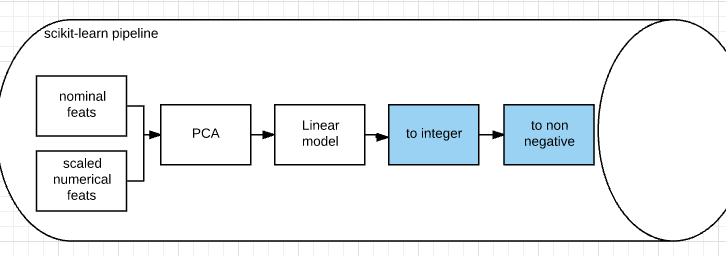An example usage is provided below:

In [ ]:
from sklearn.model_selection import GridSearchCV

param_grid = [
{"main_learning_pp__pca__n_components": [ 4, 5],
"main_learning_pp__lr__l1_ratio": [ .5,  .85, .9, .95, 1],
"main_learning_pp__lr__max_iter": ,
"main_learning_pp__lr__alpha": [ .5, .75]
}
]

gs = GridSearchCV(estimator=the_pipe,
param_grid=param_grid,
scoring=neg_rmsle_score,
cv=10,
n_jobs=1)

fitted_reg_model = gs.fit(X=reg_data_X_train, y=reg_data_Y_train.values)


# Conclusion¶

I find that removing the distinction between transform(), predict() and predict_proba() and letting everything behave like a transformer adds a lot of flexibility to the pipelines.

One direction I have note explored here is to use several models in the pipeline, combining them with FeatureUnion could easily let us designed stacked ensembles directly as scikit-learn pipelines! Given that stacking potentially augments tremendously the number of degrees of freedom of a model, it's very prone to overfitting, so it might be positive to be able to tune them as a whole, e.g. in a scikit-learn cross-validation loop. Now there is, of course, an obvious computational cost to that approach. To be digged later...